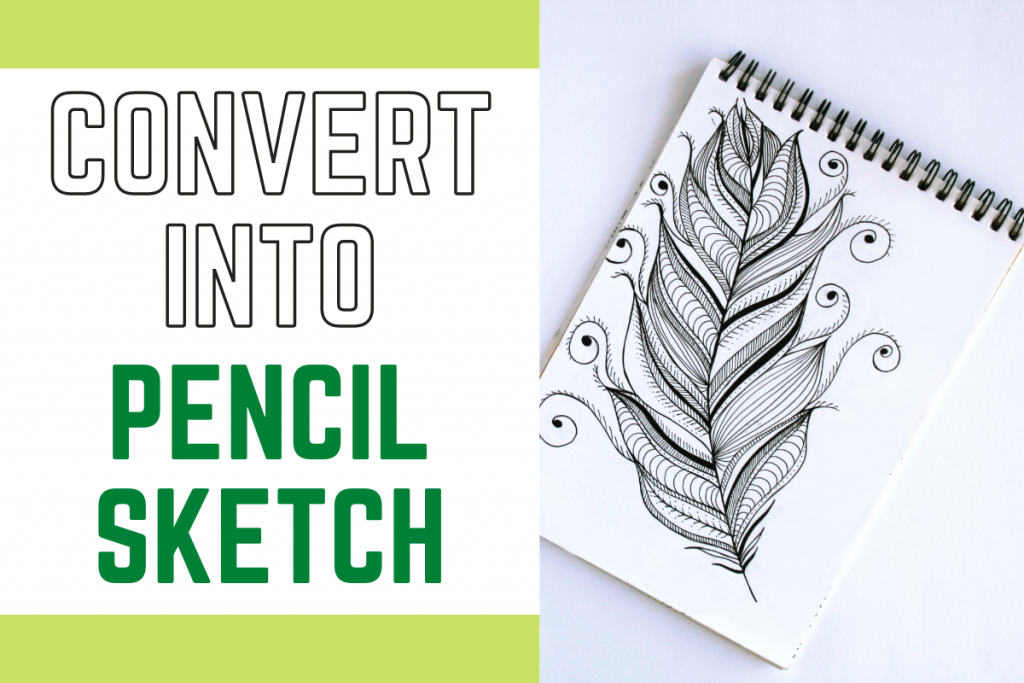# Python: Converting Images to Pencil SketchHey there! Today we are going to learn how to convert images to pencil sketches with the help of OpenCV and plot the images at various stages using matplotlib.

Recommended read: Edge detection in Python

## 1. Importing Modules

Let’s first start by importing modules into our program. We will be making use of the OpenCV functions and matplotlib module to plot the images.

We will also be setting the plotting style according to my preference.

```import cv2
import matplotlib.pyplot as plt
plt.style.use('seaborn')
```

Now we will be loading the image file into our program by making use of the `imread` function which takes the path of the file. Make sure your path to the file is correct.

We will also be changing the color code of the image to RGB format to get the original colors and plot the image using the `imshow` function.

The code for the same is shown below.

```img = cv2.imread("image1.png")
img = cv2.cvtColor(img,cv2.COLOR_BGR2RGB)
plt.figure(figsize=(8,8))
plt.imshow(img)
plt.axis("off")
plt.title("Original Image")
plt.show()
```

The image that I have been using is plotted with help of the above code and the same is displayed below.

## 3. Converting Image to GrayScale

To decrease the complexity of the image and make the handling easier we will be converting the image to a grayscale image with the help of the `cvtColor` function. The same is plotted with the help of the code mentioned below.

```img_gray = cv2.cvtColor(img, cv2.COLOR_BGR2GRAY)
plt.figure(figsize=(8,8))
plt.imshow(img_gray,cmap="gray")
plt.axis("off")
plt.title("GrayScale Image")
plt.show()
```

The output image is displayed below.

## 4. Inverting the Image

Now the next step is to invert the image. Now your question would be why to do that? The answer to the same is that when a image is inverted it helps in studying the image with more precision and detail.

The more detailed study is important for the sketch generation as the sketches need to be accurate and good. The code to invert the image is shown below.

```img_invert = cv2.bitwise_not(img_gray)
plt.figure(figsize=(8,8))
plt.imshow(img_invert,cmap="gray")
plt.axis("off")
plt.title("Inverted Image")
plt.show()
```

The output of inverted image is displayed below.

In addition to this, we will also be smoothing the image to make sure the sketch we obtain is less edgy and smooth. The code for the same is shown below.

```img_smoothing = cv2.GaussianBlur(img_invert, (21, 21),sigmaX=0, sigmaY=0)
plt.figure(figsize=(8,8))
plt.imshow(img_smoothing,cmap="gray")
plt.axis("off")
plt.title("Smoothen Image")
plt.show()
```

The output image is shown below.

## 5. Converting your images to pencil sketches

Now that the whole image processing is done, we will be passing the previous outputs to the function and pass the right and accurate parameters to make the appropriate changes to the image. The same is mentioned in the code below.

```final = cv2.divide(img_gray, 255 - img_smoothing, scale=255)
plt.figure(figsize=(8,8))
plt.imshow(final,cmap="gray")
plt.axis("off")
plt.title("Final Sketch Image")
plt.show()
```

The final output is displayed below.

## 6. The Final Output

The code below will display all the images in one frame using the subplots.

```plt.figure(figsize=(20,20))
plt.subplot(1,5,1)
plt.imshow(img)
plt.axis("off")
plt.title("Original Image")
plt.subplot(1,5,2)
plt.imshow(img_gray,cmap="gray")
plt.axis("off")
plt.title("GrayScale Image")
plt.subplot(1,5,3)
plt.imshow(img_invert,cmap="gray")
plt.axis("off")
plt.title("Inverted Image")
plt.subplot(1,5,4)
plt.imshow(img_smoothing,cmap="gray")
plt.axis("off")
plt.title("Smoothen Image")
plt.subplot(1,5,5)
plt.imshow(final,cmap="gray")
plt.axis("off")
plt.title("Final Sketch Image")
plt.show()
```

The output is displayed below.